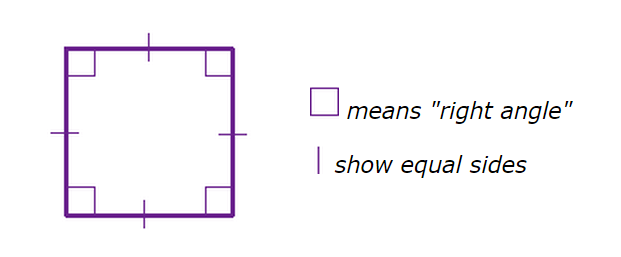Problems
416 - Side Length of Square

Side Length of Square

Time Limit: 1 sec

The Problem

A Square is a flat shape with 4 equal sides and every angle is a right angle (90°)Characteristic of Square

1. All sides are equal in length

2. Each internal angle is 90°

3. Opposite sides are parallel

The Area of square is the side length(a) squared.

Area = a2 = a x a

Write a program that read an integer number which is the area (A) of Square, your task is to find the side length of the Square.

The Input

Input file contain a series of line, each line contains only one integer numbers A , which is the area of square (0<A<=1000000). Input is terminated by EOF.

The Output

For each line of input, output will show the one integer number which is the side length of the square in a separate line.

Sample Input

100

400

25

Sample Output

10

20

5

Problem Setter: Shahin ShamS